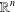Home

# A NOTE ON COMPUTING THE INTERSECTION OF SPHERES IN$\mathbb{R}^{n}$

## Abstract

Finding the intersection of$n$ -dimensional spheres in$\mathbb{R}^{n}$ is an interesting problem with applications in trilateration, global positioning systems, multidimensional scaling and distance geometry. In this paper, we generalize some known results on finding the intersection of spheres, based on QR decomposition. Our main result describes the intersection of any number of$n$ -dimensional spheres without the assumption that the centres of the spheres are affinely independent. A possible application in the interval distance geometry problem is also briefly discussed.

## References

Hide All
MathJax
MathJax is a JavaScript display engine for mathematics. For more information see http://www.mathjax.org.

# A NOTE ON COMPUTING THE INTERSECTION OF SPHERES IN$\mathbb{R}^{n}$

## Metrics

### Full text viewsFull text views reflects the number of PDF downloads, PDFs sent to Google Drive, Dropbox and Kindle and HTML full text views.

Total number of HTML views: 0
Total number of PDF views: 0 *Loading metrics...

### Abstract viewsAbstract views reflect the number of visits to the article landing page.

Total abstract views: 0 *Loading metrics...

* Views captured on Cambridge Core between <date>. This data will be updated every 24 hours.

Usage data cannot currently be displayed